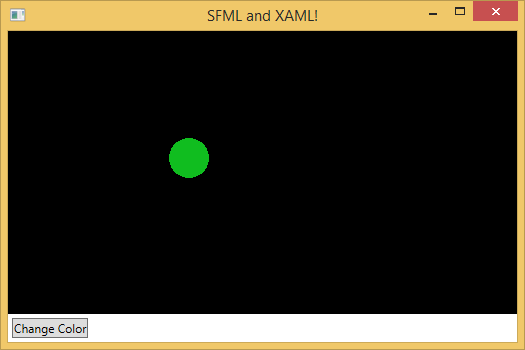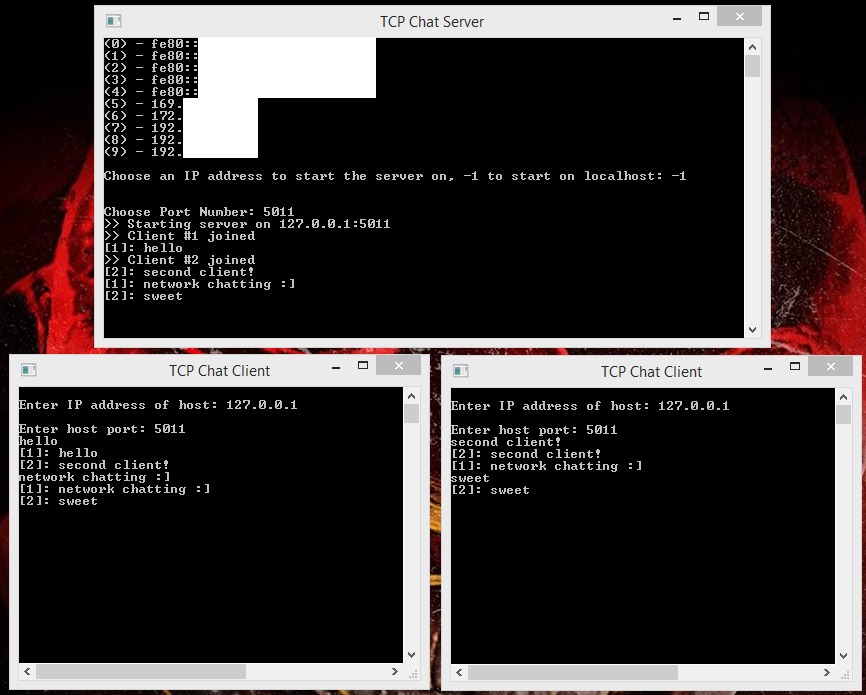# Bitmap (Pixel) Fonts

One function most games need is the ability to display information to the user using text and numbers. It’s not uncommon for graphics libraries to have support for TTF fonts (see SFML and SDL). However, given the style of game I’m working on, what I would prefer is a bitmap font.

Just type “video game bitmap fonts” into Google image search and you can see how different they are compared to TTF. If you’re making a low resolution or pixel style game then bitmap fonts really fit the theme.

I’ve found libraries that deal with TTF fonts. I’ve also found programs that will supposedly turn TTF fonts into bitmap fonts. But the fonts I want are built from the ground up as a bitmap image, and I haven’t really found any way of using bitmap fonts (with SFML .NET specifically) within a project that satisfies that.

It seemed like a decent sized task to work on myself, so I took a stab at it.

# SFML.Net and WPFI had an idea recently for a project that I both wanted to use SFML for, and wanted lots of buttons and controls. My instinct was to use SFML.Net and C#, specifically with a WPF window so I could use XAML for my layouts.

Actually getting the two to work together was harder than I expected though. But I managed to get a working setup going, which I’ll document here step-by-step.

# Matching braces in a string

I heard recently about an interview problem involving matching braces in a string. Checking to see if all the braces in a string are paired up in the proper order. Seemed like an interesting, and short, challenge.

Examples of strings with proper brace matching: "()", "(){}[]", "([{}])"

Examples of strings without proper brace matching: "(", "[]]", "([)]"

# Creating a chat roomLearning network programming has been on my backburner for quite a while now, so I decided that it was about time I got around to it.

Way back when I made a program called NetChat that was written in, of all things, a language called Blitz Basic, which was a language focused on making games. NetChat was what my friends and I used at school to chat with each other between classrooms (instead of paying attention in class…) It was single threaded and used Blitz Basic’s implementation of networking to communicate. Nonetheless it had support for multiple concurrent users, colors for users and their messages, an admin user, and even the ability for all users to draw on a picture at the same time using colored circles of various sizes.

Unfortunately the source code and program has been lost for years now, which is disappointing because it was probably the most full featured, and definitely most used, program I had made around that time. Since then I’ve been wanting to recreate it in a more common language. This post is about the first steps toward making it, simply figuring out how to send and receive pieces of data through the network.

# Factorizeroes!

A while back I was looking at the different maximum values that the different integer data types (uint8, short, int, long, etc) have throughout a couple languages I’ve been using and noticed that none of them ended in zero. I wondered why that was but then relatively quickly realized that it is because integer data types in computers are made up of bytes and bits.

An 8-bit (1-byte) integer has a max value of$2^8 = 256$, a 16-bit (2-byte) integer has a max value of$2^{16} = 65536$, etc. In fact since any integer in a computer is made of bits it will have a maximum value of$2^n$. The prime factorization of an n-bit integer is in the notation, it is n 2s all multiplied together. Like so:$2^8 = 8 \cdot 8 \cdot 8 \cdot 8 \cdot 8 \cdot 8 \cdot 8 \cdot 8$

In order for something to end in a zero it must be a multiple of 10, and the prime factorization of 10 is 5 * 2, and the prime factorization of$2^n$ will never contain a 5. Case closed. That was fun.

That got me thinking about figuring out how many zeroes are at the end of a number if all you have is the prime factorization. Using my basic arithmetic skills I found out that:

•$2 \cdot 5 = 10$
•$2 \cdot 2 \cdot 5 = 20$
•$2 \cdot 5 \cdot 5 = 50$
•$2 \cdot 2 \cdot 5 \cdot 5 = 10 \cdot 10 = 100$

It appears (although isn’t proof) that the lowest quantity between twos and fives dictates how many zeroes are at the end of a number when you’re looking at its prime factorization. I tried this with many more combinations and it worked with every one of them.

So what can we do with this information?

A factorial is a number written as$n!$ where for any value$n$,$n! = 1 \cdot 2 \cdot 3 \cdot \ldots \cdot n$ . For example$3! = 1 \cdot 2 \cdot 3$ and$5! = 1 \cdot 2 \cdot 3 \cdot 4 \cdot 5 = 120$. The Wikipedia page for factorials shows that$70! = 1.197857167 \times 10^{100}$. That’s a big number, over a googol. You can see the whole thing here on WolframAlpha.